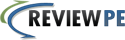No Title ID 5. FileType 9. Subtopic 10. Topic 11. Reference: 8. Minor Subject 12. Subject 6214301 Work done in moving a charge in an electric field 170 Sample Problems Complex numbers and electrostatics Complex numbers and electrostatics FERM Complex numbers and electrostatics Electric Circuits 6214302 Work done in moving a charge in an electric field 171 Sample Problems Complex numbers and electrostatics Complex numbers and electrostatics FERM Complex numbers and electrostatics Electric Circuits 6214303 Electrostatic fields 172 Sample Problems Complex numbers and electrostatics Complex numbers and electrostatics FERM Complex numbers and electrostatics Electric Circuits 6224301 Complex numbers in phasor form 173 Exam Problems Complex numbers and electrostatics Complex numbers and electrostatics FERM Complex numbers and electrostatics Electric Circuits 6224302 Work required to move a charge 174 Exam Problems Complex numbers and electrostatics Complex numbers and electrostatics FERM Complex numbers and electrostatics Electric Circuits 6224303 Phasor operations 175 Exam Problems Complex numbers and electrostatics Complex numbers and electrostatics FERM Complex numbers and electrostatics Electric Circuits 6224305 Coulomb's law 176 Exam Problems Complex numbers and electrostatics Complex numbers and electrostatics FERM Complex numbers and electrostatics Electric Circuits 6224402 Simple circuit 180 Exam Problems Complex numbers and electrostatics Complex numbers and electrostatics FERM Complex numbers and electrostatics Electric Circuits